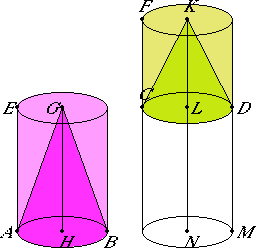# Proposition 14

Cones and cylinders on equal bases are to one another as their heights.

Let EB and FD be cylinders on equal bases, the circles AB and CD.

I say that the cylinder EB is to the cylinder FD as the axis GH is to the axis KL.I.3

Produce the axis KL to the point N, make LN equal to the axis GH, and construct the cylinder CM about LN as the axis.

XII.11

Then, since the cylinders EB and CM are of the same height, therefore they are to one another as their bases.

But the bases equal one another, therefore the cylinders EB and CM are also equal.

XII.13

And, since the cylinder FM has been cut by the plane CD parallel to its opposite planes, therefore the cylinder CM is to the cylinder FD as the axis LN is to the axis KL.

But the cylinder CM equals the cylinder EB, and the axis LN equals the axis GH, therefore the cylinder EB is to the cylinder FD as the axis GH is to the axis KL.

XII.10

But the cylinder EB is to the cylinder FD as the cone ABG is to the cone CDK. Therefore the axis GH is to the axis KL as the cone ABG is to the cone CDK and as the cylinder EB is to the cylinder FD.

Therefore, cones and cylinders on equal bases are to one another as their heights.

Q.E.D.

## Guide

Back in proposition XII.11, cones and cylinders were shown to be proportional to their bases, and this proposition shows that they are proportional to their heights. The next proposition relates the volume to the base and height in a different way by fixing the volume so that the base and height are reciprocally proportional.